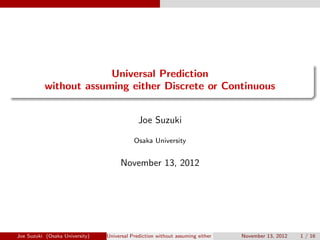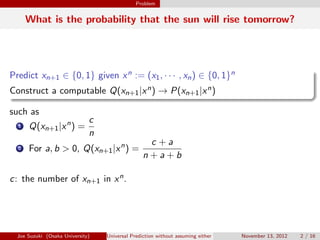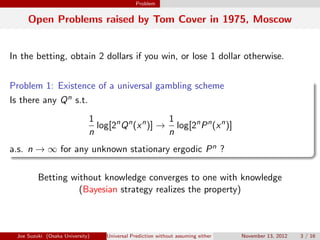Successfully reported this slideshow.

# Universal Prediction without assuming either Discrete or Continuous

0

Share×
1 of 16
1 of 16

# Universal Prediction without assuming either Discrete or Continuous

0

Share

Joe Suzuki, Universal Prediction without assuming either Discrete or Continuous, November 2012

Joe Suzuki, Universal Prediction without assuming either Discrete or Continuous, November 2012

## More Related Content

### Related Books

Free with a 14 day trial from Scribd

See all

### Related Audiobooks

Free with a 14 day trial from Scribd

See all

### Universal Prediction without assuming either Discrete or Continuous

1. 1. . . Universal Prediction without assuming either Discrete or Continuous Joe Suzuki Osaka University November 13, 2012 Joe Suzuki (Osaka University) Universal Prediction without assuming either Discrete or ContinuousNovember 13, 2012 1 / 16
2. 2. Problem What is the probability that the sun will rise tomorrow? Predict xn+1 ∈ {0, 1} given xn := (x1, · · · , xn) ∈ {0, 1}n . . Construct a computable Q(xn+1|xn) → P(xn+1|xn) such as 1 Q(xn+1|xn ) = c n 2 For a, b > 0, Q(xn+1|xn ) = c + a n + a + b   c: the number of xn+1 in xn. Joe Suzuki (Osaka University) Universal Prediction without assuming either Discrete or ContinuousNovember 13, 2012 2 / 16
3. 3. Problem Open Problems raised by Tom Cover in 1975, Moscow In the betting, obtain 2 dollars if you win, or lose 1 dollar otherwise.   Problem 1: Existence of a universal gambling scheme . Is there any Qn s.t. 1 n log[2n Qn (xn )] → 1 n log[2n Pn (xn )] a.s. n → ∞ for any unknown stationary ergodic Pn ? Betting without knowledge converges to one with knowledge (Bayesian strategy realizes the property) Joe Suzuki (Osaka University) Universal Prediction without assuming either Discrete or ContinuousNovember 13, 2012 3 / 16
4. 4. Problem Problem 2: Existence of a universal prediction scheme . . Is there any Q s.t. for x ∈ {0, 1} Q(x|x−1 −n ) → P(x|x−1 −∞) a.s. n → ∞ for any unknown stationary ergodic P ? Ornstein 1978 (discrete, Non-Bayesian) Algoet 1992 (extended to the Polish spaces, Non-Bayesian) x−1 −∞ ∈ {0, 1}∞ → ({sk}, {tk}), s0 < s1 < · · · , t0 < t1 < · · · s.t. Q(x|x−1 −tk ) = #Ik(x) + 1/2 #Ik(0) + #Ik(1) + 1 Ik(x) = {1 ≤ τ ≤ sk|x = x−τ , x−1 −tk = x−τ−1 −τ−tk } Joe Suzuki (Osaka University) Universal Prediction without assuming either Discrete or ContinuousNovember 13, 2012 4 / 16
5. 5. Problem Bayesian for binary i.i.d. sources Qn (xn ) = ∫ w(θ)P(xn |θ)dθ , P(xn |θ) = θc (1 − θ)n−c For a, b > 0, w(θ) ∝ θ−a (1 − θ)−b ⇐⇒ Q(xn+1|xn ) = Qn+1(xn+1) Qn(xn) = c + a n + a + b For a = b = 1/2 (Krichevsky-Troﬁmov), − 1 n log Qn (xn ) → H := ∑ x∈A −P(x) log P(x) − 1 n log Pn (xn ) = 1 n n∑ i=1 − log P(xi ) → E[− log P(xi )] = H Joe Suzuki (Osaka University) Universal Prediction without assuming either Discrete or ContinuousNovember 13, 2012 5 / 16
6. 6. Problem Universality There exists Qn s.t. for any Pn 1 Q(x|x−1 −n ) → P(x|x−1 −∞) (1) 2 1 n log Pn(xn) Qn(xn) → 0 (2) m-nary (m ≥ 2) rather than binary stationary ergodic rather than i.i.d. Ornstein 1978 (1) Bayesian (2) as well as (1) Joe Suzuki (Osaka University) Universal Prediction without assuming either Discrete or ContinuousNovember 13, 2012 6 / 16
7. 7. Problem Problem Construct Qn satisfying (2) for the genaral case . . Xn should be stationary ergodic but can be either discrete, continuous, or neither of them Counting how many (X = xi+1, Xi = xi ) occurs does not help. Algoet 1992 does not imply (2) for the general case. Joe Suzuki (Osaka University) Universal Prediction without assuming either Discrete or ContinuousNovember 13, 2012 7 / 16
8. 8. Density Functions Suppose a density function f exists for X A: the range of X A0 := {A} Aj+1 is a reﬁnement of Aj Example 1: Quantize f over A = [0, 1) to obtain histogram approximations f1 over A1 = {[0, 1/2), [1/2, 1)} f2 over A2 = {[0, 1/4), [1/4, 1/2), [1/2, 3/4), [3/4, 1)} . . . fj over Aj = {[0, 2−(j−1)), [2−(j−1), 2 · 2−(j−1)), · · · , [(2j−1 − 1)2−(j−1), 1)} . . . Pn j (an) = ∏n i=1 Pj (ai ), the probability of an = (a1, · · · , an) ∈ An j Qn j : a Bayesian measure 1 n log Pn j (an) Qn j (an) → 0 as n → ∞ Joe Suzuki (Osaka University) Universal Prediction without assuming either Discrete or ContinuousNovember 13, 2012 8 / 16
9. 9. Density Functions λ : R → B (Lebesgue measure, a = [b, c) =⇒ λ(a) = c − b) (x1, · · · , xn) ∈ (a1, · · · , an) ∈ An j =⇒    f n j (xn ) := fj (x1) · · · fj (xn) = Pj (a1) · · · Pj (an) λ(a1) . . . λ(an) gn j (xn ) := Qn j (a1, · · · , an) λ(a1) · · · λ(an) For {ωj }∞ j=1: ∑ ωj = 1, ωj > 0, gn (xn ) := ∞∑ j=1 ωj gn j (xn ) If we choose {Aj } such that fj → f as j → ∞, for any f , almost surely 1 n log f n(xn) gn(xn) → 0 (3) B. Ryabko. IEEE Trans. on Inform. Theory, 55, 9, 2009. Joe Suzuki (Osaka University) Universal Prediction without assuming either Discrete or ContinuousNovember 13, 2012 9 / 16
10. 10. Generalized Density Functions Exactly when does density function exist? B: the Borel sets of R µ(D): the probabbility of D ∈ B When a density function exists . The following are equivalent (µ ≪ λ): for each D ∈ B, λ(D) = 0 =⇒ µ(D) = 0 ∃ B-measurable dµ dλ := f s.t. µ(D) = ∫ D f (t)dλ(t) Joe Suzuki (Osaka University) Universal Prediction without assuming either Discrete or ContinuousNovember 13, 2012 10 / 16
11. 11. Generalized Density Functions Estimating generalized density functions Radon-Nikodym’s Theorem . . The following are equivalent (µ ≪ η): for each D ∈ B, η(D) = 0 =⇒ µ(D) = 0 ∃ B-measurable dµ dη := f s.t. µ(D) = ∫ D f (t)dη(t) Example 2: µ({k}) > 0, η({k}) := 1 k(k + 1) , k ∈ B := {1, 2, · · · } µ(D) = ∑ k∈D f (k)η({k}) , D ⊆ B µ ≪ η =⇒ dµ dη (k) = f (k) = µ({k}) η({k}) = k(k + 1)µ({k}) Joe Suzuki (Osaka University) Universal Prediction without assuming either Discrete or ContinuousNovember 13, 2012 11 / 16
12. 12. Generalized Density Functions f1 over B1 := {{1}, {2, 3, · · · }} f2 over B2 := {{1}, {2}, {3, 4, · · · }} . . . fk over Bk := {{1}, {2}, · · · , {k}, {k + 1, k + 2, · · · }} . . . (y1, · · · , yn) ∈ (b1, · · · , bn) ∈ Bn k =⇒ gn k (yn ) := Qn k (b1, · · · , bn) η(b1) · · · η(bn) gn (yn ) := ∞∑ k=1 ωkgn k (yn ) If we choose {Bk} s.t. fk → f , for any f , almost surely 1 n log f n(yn) gn(yn) → 0 (4) gn(yn) ∏n i=1 ηn({yi }) estimates P(yn) = f n(yn) ∏n i=1 ηn({yi }) Joe Suzuki (Osaka University) Universal Prediction without assuming either Discrete or ContinuousNovember 13, 2012 12 / 16
13. 13. Generalized Density Functions The original case was contained as a special case For C = {0, 1, · · · , m − 1}, if we quantize C1 = C2 = · · · = {{0}, {1}, · · · , {m − 1}} η({0}) = · · · η({m − 1}) = 1/m then µ ≪ η and zn ∈ Cn ⇐⇒ cn ∈ Cn 1 = Cn 2 = · · · =⇒    f n (zn ) = Pn(cn) (1/m)n , gn 1 (zn ) = gn 2 (zn ) = · · · = gn (zn ) = ∞∑ l=1 ωl gn l (zn ) = Qn(cn) (1/m)n =⇒ 1 n log f n(zn) gn(zn) = 1 n log Pn(cn) Qn(cn) → 0 Joe Suzuki (Osaka University) Universal Prediction without assuming either Discrete or ContinuousNovember 13, 2012 13 / 16
14. 14. The Solution Universality in the generalized sense If µn ≪ ηn, there exists gn without depending on f n s.t. 1 n log f n(zn) gn(zn) → 0 µn (Dn ) := ∫ D f n (zn )dηn (zn ) , νn (Dn ) := ∫ D gn (zn )dηn (zn ) f n(zn) gn(zn) = dµn dηn (zn )/ dνn dηn (zn ) = dµn dνn (zn ) Theorem (Suzuki, 2011) 1 n log dµn dνn (zn ) → 0 Joe Suzuki (Osaka University) Universal Prediction without assuming either Discrete or ContinuousNovember 13, 2012 14 / 16
15. 15. The Solution Universal Prediction in the generalized sense The generalzed universal density function tells everything: g(xn+1|xn ) = gn+1(xn+1) gn(xn) → f (xn+1|xn ) = f n+1(xn+1) f n(xn)   For any D ∈ B, ν(D|xn ) = ∫ D g(x|xn )dη(x) Joe Suzuki (Osaka University) Universal Prediction without assuming either Discrete or ContinuousNovember 13, 2012 15 / 16
16. 16. Summary Summary and Discussion Universal Prediction . . Connection to Universal Bayesian Measures Generalization without assuming Discrete or Continuous Stronger universality in the sense of Bayes. Many Applications except Prediction Bayesian network structure estimation (DCC 2012) The Bayesian Chow-Liu Algorithm (PGM 2012) Markov order estimation even when {Xi } is continuous Joe Suzuki (Osaka University) Universal Prediction without assuming either Discrete or ContinuousNovember 13, 2012 16 / 16